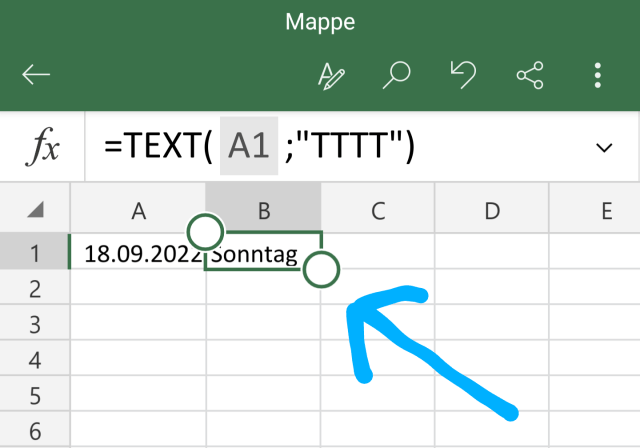# Excel How to Calculate and display the day of the week from the date?

If you use Excel and want to display the day of the week for a certain date, it is very easy with the right formula. We would like to explain how you can easily calculate and display the day of the week from a date.

## How to calculate the day of the week for a date in ExcelExample:

 Column A Column B 18.09.2022 Weekday??

You now want to display the day of the week in column B for the date in column A. Select the cell in column B and enter the following code at the top of the formula line:

• =TEXT(A1; "DDD")

The Excel code explains:

• The "TEXT" command formats the cell as text.
• A1 defines the reference to column A cell 1
• TTTT defines that you want the day of the week to be displayed.

You now get the following result:

 Column A Column B 18.09.2022 Sunday??

The calculation is done by Excel in the background, where a perpetual calendar is stored. This means that you can have the corresponding weekday displayed for a date at any time. Not practical?

Now you know the very simple way to generate a weekday from a date in Excel.